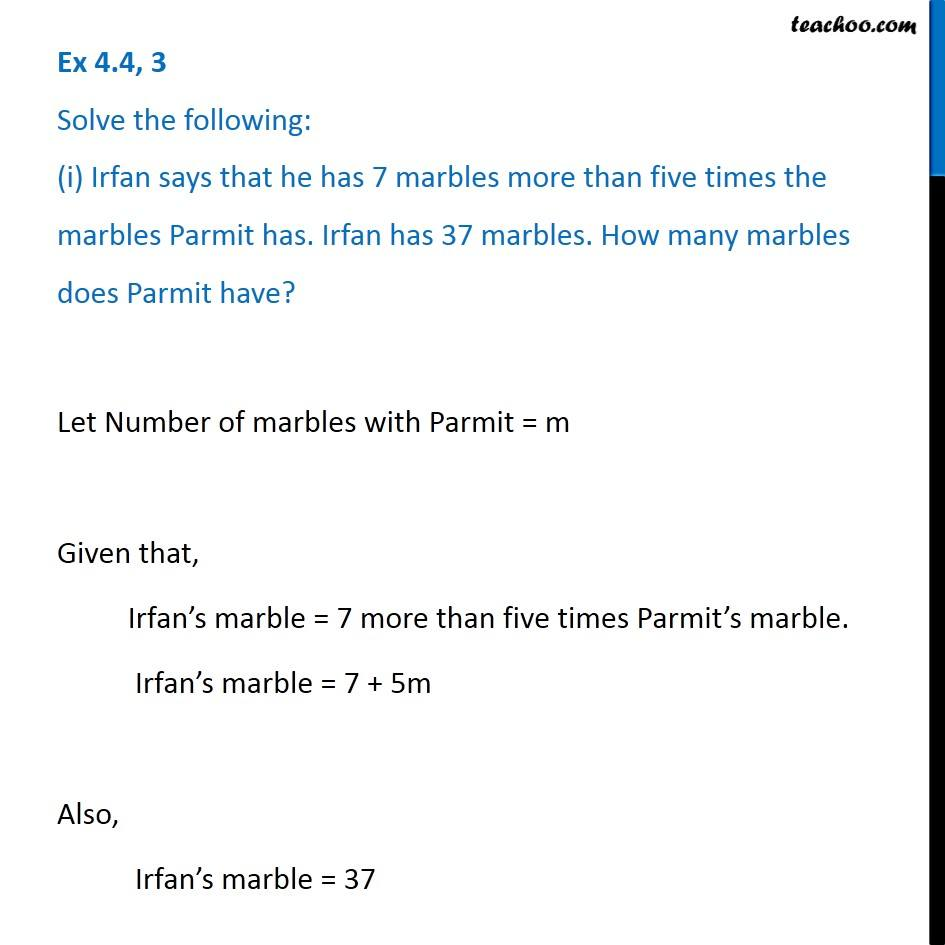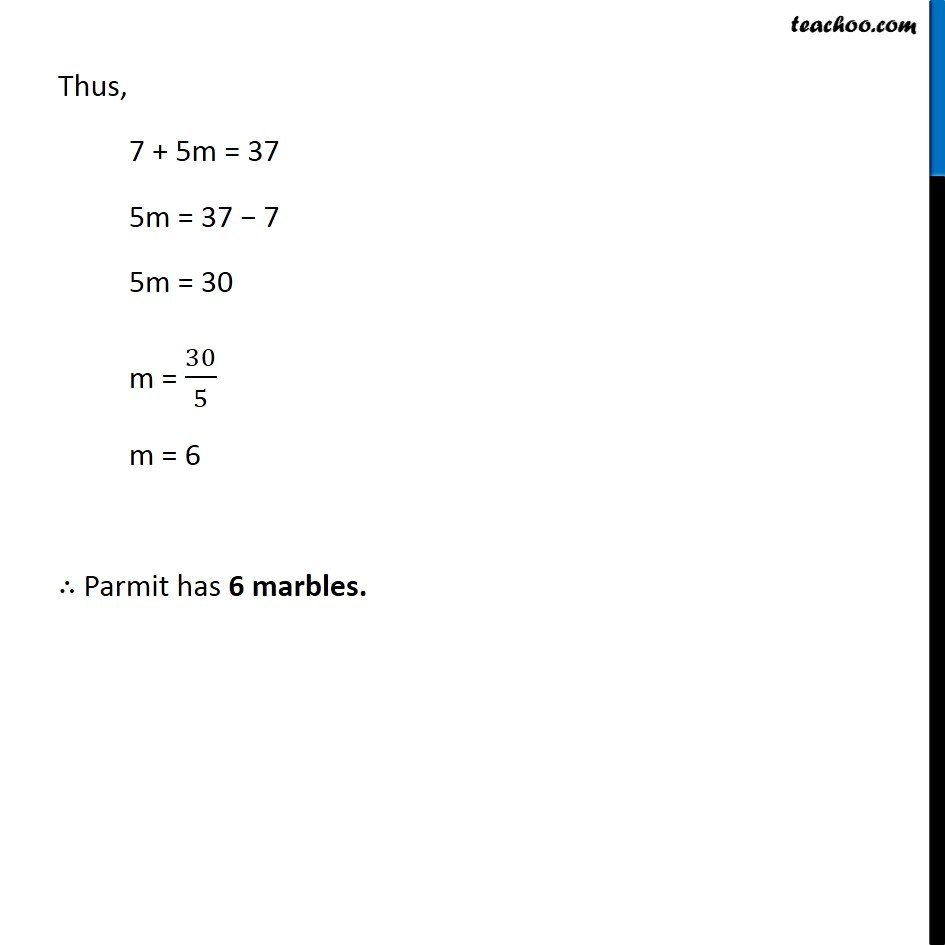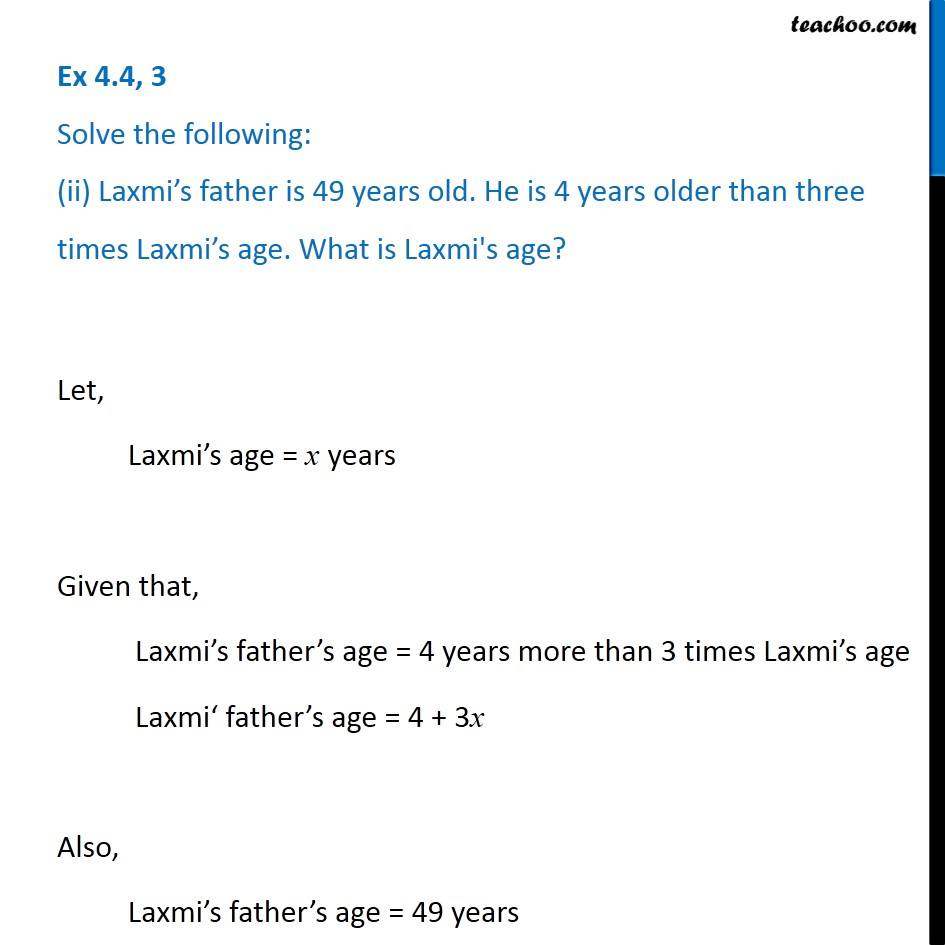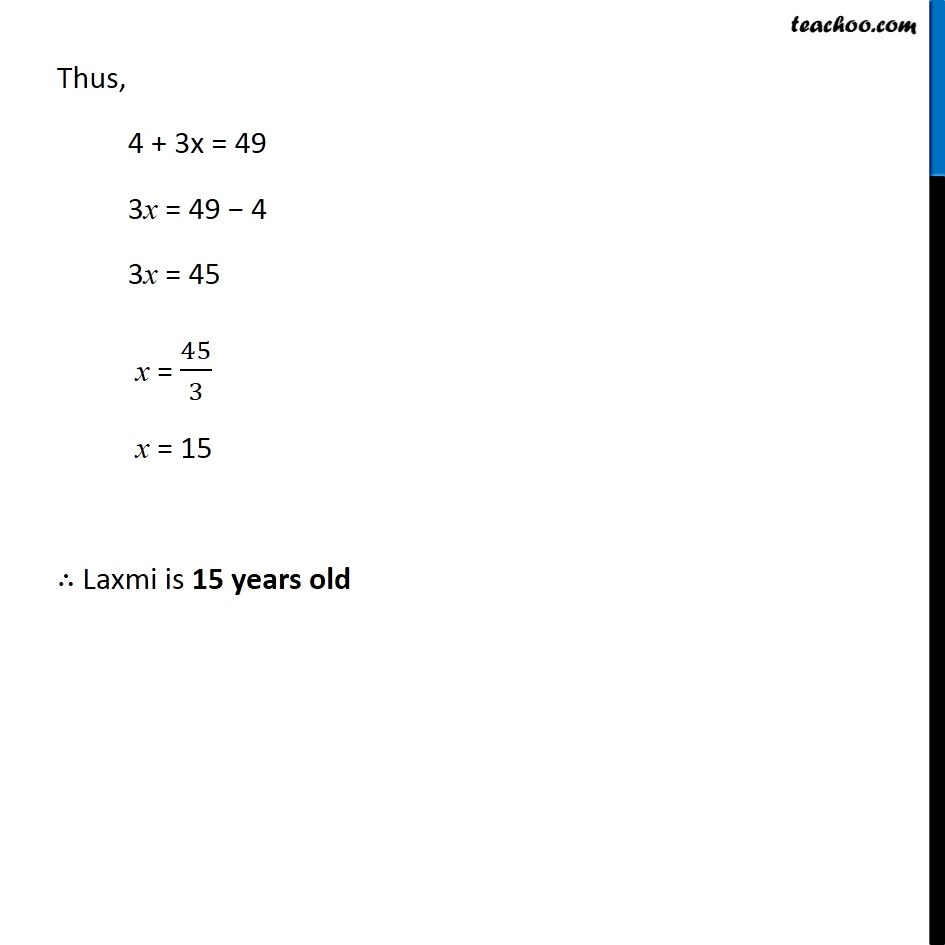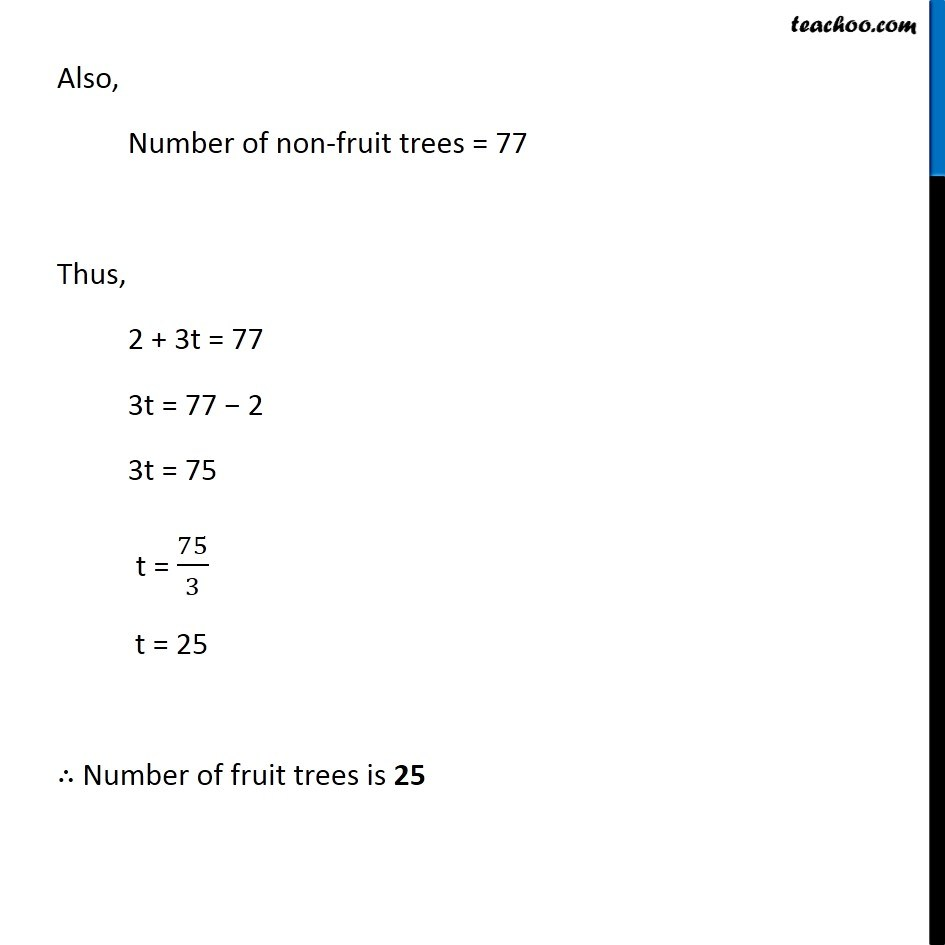Subscribe to our Youtube Channel - https://you.tube/teachoo

1. Chapter 4 Class 7 Simple Equations
2. Serial order wise
3. Ex 4.4

Transcript

Ex 4.4, 3 Solve the following: (i) Irfan says that he has 7 marbles more than five times the marbles Parmit has. Irfan has 37 marbles. How many marbles does Parmit have?Let Number of marbles with Parmit = m Given that, Irfan’s marble = 7 more than five times Parmit’s marble. Irfan’s marble = 7 + 5m Also, Irfan’s marble = 37 Thus, 7 + 5m = 37 5m = 37 − 7 5m = 30 m = 30/5 m = 6 ∴ Parmit has 6 marbles. Ex 4.4, 3 Solve the following: (ii) Laxmi’s father is 49 years old. He is 4 years older than three times Laxmi’s age. What is Laxmi's age?Let, Laxmi’s age = x years Given that, Laxmi’s father’s age = 4 years more than 3 times Laxmi’s age Laxmi‘ father’s age = 4 + 3x Also, Laxmi’s father’s age = 49 years Thus, 4 + 3x = 49 3x = 49 − 4 3x = 45 x = 45/3 x = 15 ∴ Laxmi is 15 years old Ex 4.4, 3 Solve the following: (iii) People of Sundargram planted trees in the village garden. Some of the trees were fruit trees. The number of non-fruit trees were two more than three times the number of fruit trees. What was the number of fruit trees planted if the number of non-fruit trees planted was 77?Let number of fruit of fruit trees = t Given that, Number of Non-fruit trees = 2 more than 3 times the number of fruit trees Number of non-fruit trees = 2 + 3t. Also, Number of non-fruit trees = 77 Thus, 2 + 3t = 77 3t = 77 − 2 3t = 75 t = 75/3 t = 25 ∴ Number of fruit tr

Ex 4.4